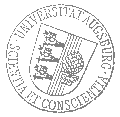# Universität AugsburgInstitut für MathematikAugsburger Mathematisches Kolloquium

Dr. Enno Keßler
Max-Planck Institut für Mathematik, Bonn

spricht am

Dienstag, 22. November 2022

um

17:30 Uhr

im

Raum 2004 (L1)

über das Thema:

## »Supergeometric Generalizations of Harmonic Maps and J-holomorphic Curves«

 Abstract: The Dirichlet Action Functional for maps from a surface to a Riemannian target manifold is conformally invariant. Hence it can be used as a tool to study the Teichmüller space of complex structures on the surface. Furthermore, if the target manifold is almost Kähler J-holomorphic curves are an interesting class of minimizers of the Dirichlet action with many important applications in symplectic geometry. The two-dimensional non-linear supersymmetric sigma model is an extension of the Dirichlet action by spinorial fields. Its supersymmetry properties require that the spinorial fields are anti-commutative. In this talk, I want to show how geometry can be extended to supergeometry which contains dimensions with anti-commuting coordinates and motivate supergeometric generalizations of Riemann surfaces, harmonic maps and J-holomorphic curves. This yields a supergeometric understanding of the two-dimensional non-linear supersymmetric sigma model, its symmetries and critical points.

 Hierzu ergeht herzliche Einladung. Prof. Dr. Maxim Smirnov

Kaffee, Tee und Gebäck eine halbe Stunde vor Vortragsbeginn im Raum 2006 (L1).

[Impressum]      [Datenschutz]      wwwadm@math.uni-augsburg.de,     Mo 14-Nov-2022 08:53:57 MEZ